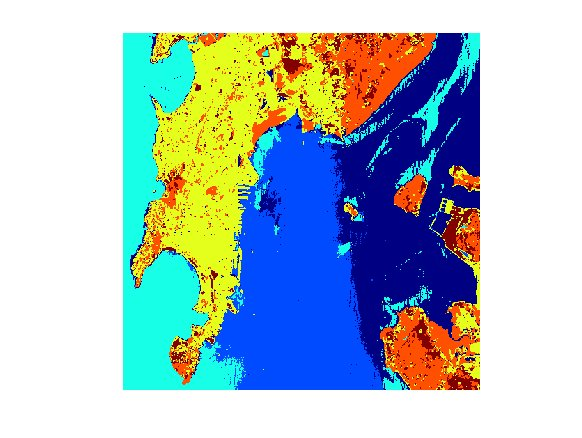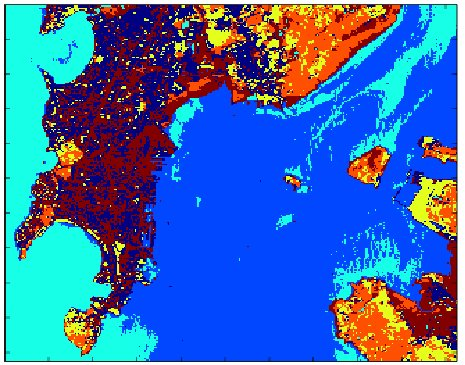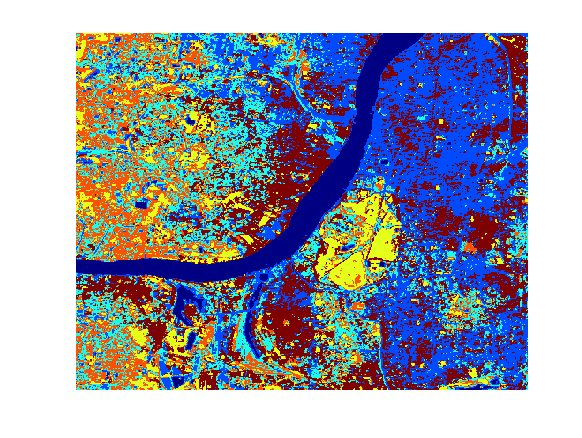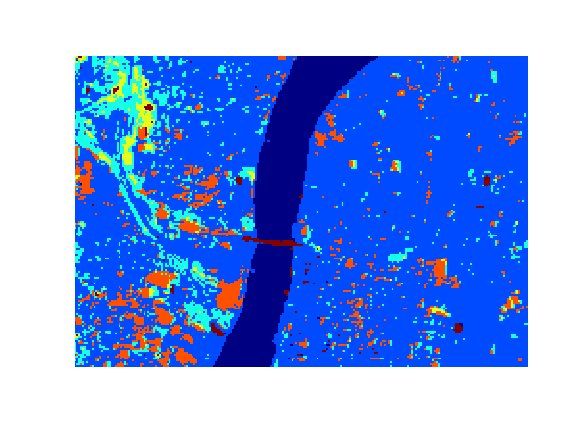CTYPE HTML PUBLIC "-//W3C//DTD HTML 4.0 Transitional//EN"> AMOSA: Archived MultiObjective Simulated Annealing (Version 2)

• Developed one Multiobjective Simulated Annealing Algorithm, AMOSA (paper has been published in IEEE Transactions on Evolutionary Computation). The program is being updated. Download a preliminary version of the program from here.

Note that the code should not be used for any business purpose.

FORM

If you use this software, please quote the following reference:

Sanghamitra Bandyopadhyay, Sriparna Saha, Ujjwal Maulik and Kalyanmoy Deb. A Simulated Annealing Based Multi-objective Optimization Algorithm: AMOSA , IEEE Transactions on Evolutionary Computation, Volume 12, No. 3, JUNE 2008, Pages 269-283.

Results of MCVGAPS Clustering Technique accepted in:

Sriparna Saha and Sanghamitra Bandyopadhyay. Application of a Multiseed Based Clustering Technique for Automatic Satellite Image Segmentation , IEEE Geoscience and Remote Sensing Letters, (accepted).

Some Literature Studies (not included in the final paper due to page restriction):

Genetic algorithms (GAs) are popularly used in pixel classification. A context-sensitive clustering technique for unsupervised image segmentation based on graph-cut initialization and expectation-maximization algorithm is developed in . An unsupervised hyperspectral image classification technique based on fuzzy-clustering algorithms that spatially exploit membership relations is proposed in . A multiresolution remote sensing image clustering technique is proposed in  which uses information contained in both spatial resolutions. An agglomerative hierarchical clustering method for large multispectral images, which uses both spectral and spatial information for the aggregation decision, is proposed in . A new spectral-spatial classification scheme for hyperspectral images which combines the results of a pixel wise support vector machine classification and the segmentation map obtained by partitional clustering using majority voting is proposed in . Recently, a multiobjective fuzzy genetic clustering technique for pixel classification has been proposed in .

 M. Tyagi, F. Bovolo, A. Mehra, S. Chaudhuri, and L. Bruzzone, A context-sensitive clustering technique based on graph-cut initialization and expectation-maximization algorithm, IEEE Geoscience and Remote Sensing Letters, vol. 5, no. 1, pp. 21 25, January 2008.

 G. Bilgin, S. Erturk, and T. Yildirim, Unsupervised classification of hyperspectral-image data using fuzzy approaches that spatially exploit membership relations, IEEE Geoscience and Remote Sensing Letters, vol. 5, no. 4, pp. 673 677, October 2008.

 C. Wemmert, A. Puissant, G. Forestier, and P. Gancarski, Multireso- lution remote sensing image clustering, IEEE Geoscience and Remote Sensing Letters, vol. 6, no. 3, pp. 533 537, July, 2009.

 A. Marcal and L. Castro, Hierarchical clustering of multispectral images using combined spectral and spatial criteria, IEEE Geoscience and Remote Sensing Letters, vol. 2, no. 1, pp. 59 63, January 2005.

 Y. Tarabalka, J. A. Benediktsson, and J. Chanussot, Spectralspatial classification of hyperspectral imagery based on partitional clustering techniques, IEEE Transactions on Geoscience and Remote Sensing, vol. 47, no. 8, pp. 29732987, Aug., 2009.

 S. Bandyopadhyay, U. Maulik, and A. Mukhopadhyay, Multiobjective genetic clustering for pixel classification in remote sensing imagery, IEEE Transactions on Geoscience and Remote Sensing, vol. 45, no. 5, pp. 1506 1511, May, 2007.

Complexity analysis of MCVGAPS Clustering Technique:

Below we have analyzed the complexity of the proposed MCVGAPS clustering.

1) Initialization of GA needs Popsize* stringlength time where Popsize and stringlength indicate the population size and the length of each chromosome in the GA respectively. Note that for maximum Kmax clusters with maximum Cmax number of sub-cluster centers per cluster in d dimensional space, stringlength will become Kmax* Cmax* d.

2) For assignment of points to different clusters total time needed is O(n * Kmax * Cmax) for each chromosome. Merging of centers will take O((Kmax *Cmax) ^2) time. Fitness is computed in O(n log n) time (this is due to point symmetry distance computation in Sym-index). So the fitness evaluation has total complexity=O(Popsize* nlog n) (assuming n >> Kmax * Cmax).

3) Selection step of the GA requires O(Popsize * stringlength) time.

4) Mutation and Crossover require O(Popsize * stringlength) time each.

So in general total time complexity becomes O(nlogn* Popsize) per generation. For maximum maxgen number of generations total complexity becomes O(nlogn* Popsize * maxgen).

Results

IRS Image of Mumbai:

The IRS image of Mumbai was obtained using the LISS-II sensor, available in four bands, viz., blue, green, red and near infra-red. Following figure shows the IRS image of a part of Mumbai in the near infra red band.The segmentation automatically identified by the proposed MCVGAPS clustering technique is shown in following Figure.Number of centers per clusters automatically evolved by MCVGAPS clustering technique are 6, 4, 3, 4, 3 and 5, respectively. It can be seen from the figure that the water (Arabian sea) surrounding Mumbai gets differentiated into three distinct regions, based on the difference in their spectral properties. The islands, dockyard have mostly been correctly identified in the image. GAPS-clustering with Sym-index gets its optimal value for K=6. MCVGAPS clustering with PS-index, I-index and XB-index as objective functions get their optimum values for K=6, K=5 and K=3, respectively. Following figure demonstrates the Mumbai image clustered using the FCM technique when K=6 is given a priori.Subtractive clustering technique automatically yields K=4 clusters from this data set. In order to show the effectiveness of MCVGAPS clustering technique quantitatively, I index and XB-index values are again calculated. I index values obtained by the MCVGAPS, FCM, GAPS with Sym-index and Subtractive clustering techniques are 205.36, 23.057, 180.45 and 60.65, respectively. Similarly XB-index values obtained by the MCVGAPS, FCM, GAPS with Sym-index and Subtractive clustering techniques are 1.028, 4.67, 2.9 and 9.15, respectively. Each algorithm is executed five times for these image data sets and the best values over these five runs are reported here. Smaller value of XB-index and larger value of I index correspond to good clustering. The values again establish the effectiveness of MCVGAPS clustering technique. The values again show that the segmentation provided by MCVGAPS clustering is much better than those of FCM-clustering, GAPS clustering optimizing Sym-index and subtractive clustering technique.

SPOT Image of Kolkata:

See paper. Some more figures are enumerated below:    The segmentation result obtained by Fuzzy C-means algorithm on this image for K=6 is shown in following Figure.Bridge detected by MCVGAPS cluststreing technique: